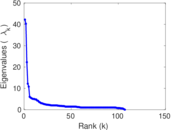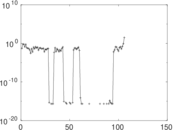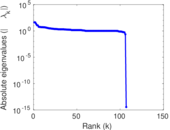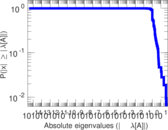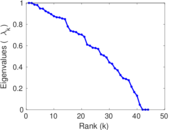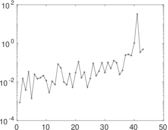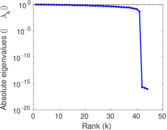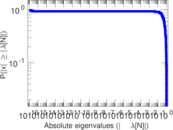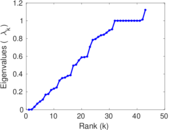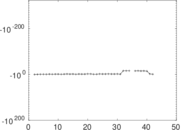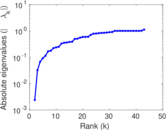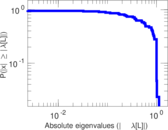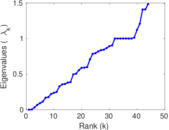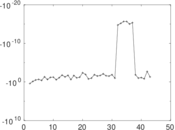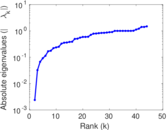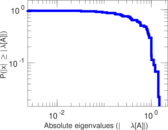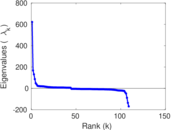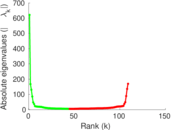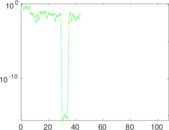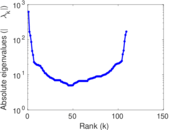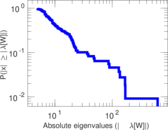# Wikiquote edits (ang)

This is the bipartite edit network of the Old English Wikiquote. It contains users and pages from the Old English Wikiquote, connected by edit events. Each edge represents an edit. The dataset includes the timestamp of each edit.

 Code `qang` Internal name `edit-angwikiquote` Name Wikiquote edits (ang) Data source http://dumps.wikimedia.org/ AvailabilityDataset is available for download Consistency checkDataset passed all tests Category Authorship network Dataset timestamp 2017-10-20 Node meaning User, article Edge meaning Edit Network formatBipartite, undirected Edge typeUnweighted, multiple edges Temporal dataEdges are annotated with timestamps

## Statistics

 Size n = 625 Left size n1 = 109 Right size n2 = 516 Volume m = 1,303 Unique edge count m̿ = 823 Wedge count s = 56,787 Claw count z = 4,034,905 Cross count x = 224,438,420 Square count q = 21,264 4-Tour count T4 = 400,706 Maximum degree dmax = 432 Maximum left degree d1max = 432 Maximum right degree d2max = 42 Average degree d = 4.169 60 Average left degree d1 = 11.954 1 Average right degree d2 = 2.525 19 Fill p = 0.014 632 7 Average edge multiplicity m̃ = 1.583 23 Size of LCC N = 446 Diameter δ = 14 50-Percentile effective diameter δ0.5 = 3.237 39 90-Percentile effective diameter δ0.9 = 8.503 37 Median distance δM = 4 Mean distance δm = 4.900 59 Gini coefficient G = 0.661 897 Balanced inequality ratio P = 0.251 727 Left balanced inequality ratio P1 = 0.142 748 Right balanced inequality ratio P2 = 0.354 566 Relative edge distribution entropy Her = 0.795 138 Power law exponent γ = 3.335 10 Tail power law exponent γt = 2.051 00 Tail power law exponent with p γ3 = 2.051 00 p-value p = 0.031 000 0 Left tail power law exponent with p γ3,1 = 1.881 00 Left p-value p1 = 0.340 000 Right tail power law exponent with p γ3,2 = 5.261 00 Right p-value p2 = 0.000 00 Degree assortativity ρ = −0.124 579 Degree assortativity p-value pρ = 0.000 340 374 Spectral norm α = 42.201 9 Algebraic connectivity a = 0.002 382 96 Spectral separation |λ1[A] / λ2[A]| = 1.048 35 Controllability C = 409 Relative controllability Cr = 0.658 615

## Plots

### Fruchterman–Reingold graph drawing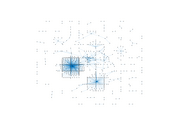### Degree distribution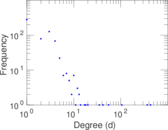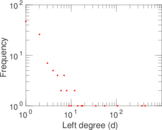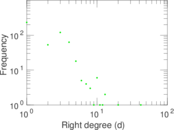### Cumulative degree distribution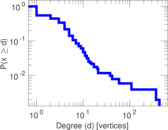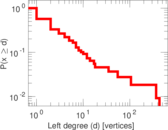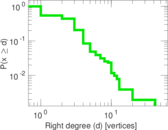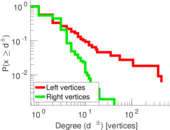### Lorenz curve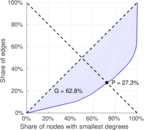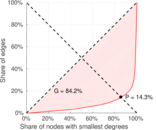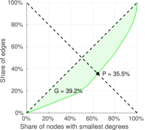### Spectral distribution of the adjacency matrix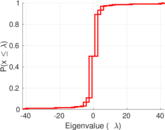### Spectral distribution of the normalized adjacency matrix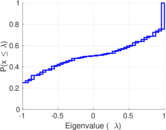### Spectral distribution of the Laplacian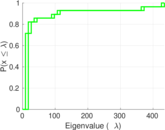### Spectral graph drawing based on the adjacency matrix### Spectral graph drawing based on the Laplacian### Spectral graph drawing based on the normalized adjacency matrix### Degree assortativity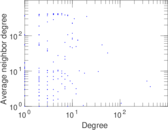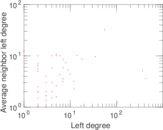### Zipf plot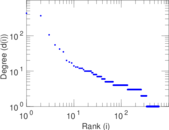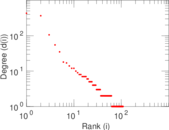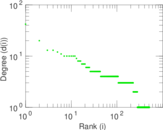### Hop distribution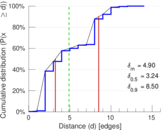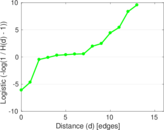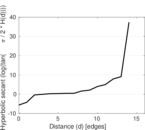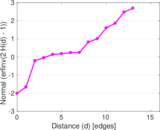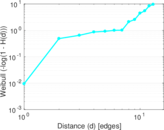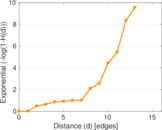### Double Laplacian graph drawing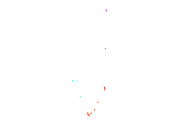### Delaunay graph drawing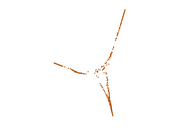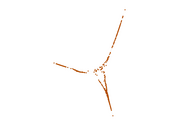### Edge weight/multiplicity distribution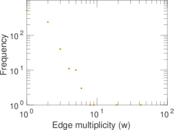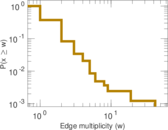### Temporal distribution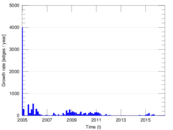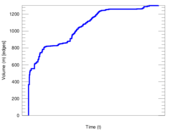### Temporal hop distribution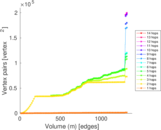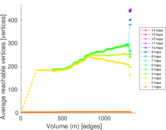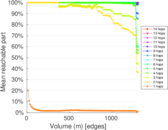### Diameter/density evolution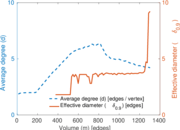### Matrix decompositions plots